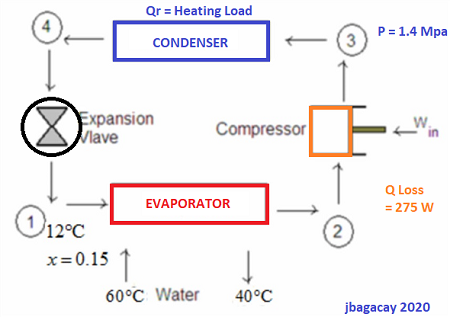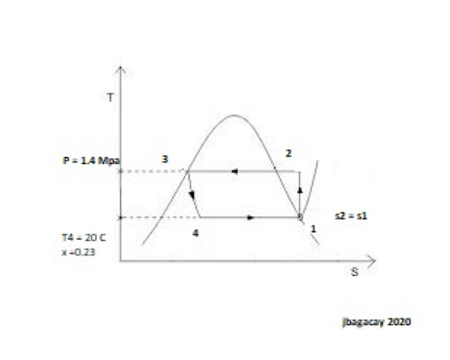# A heat pump with refrigerant R-134A, as the working fluid is used to keep a space at 25 Degree C...

## Question:

A heat pump with refrigerant {eq}R-134A{/eq}, as the working fluid is used to keep a space at {eq}25^oC{/eq} by absorbing heat from geothermal water that enters the evaporator at {eq}50^oC{/eq} at a rate of {eq}0.054\enspace kg/s{/eq} and leaves at {eq}40^oC{/eq}. The refrigerant enters the evaporator at {eq}20^oC{/eq} with a quality of 23% and leaves at the inlet pressure as saturated vapor. The refrigerant loses {eq}275 W{/eq} of heats to the surroundings as it flows through the compressor and the refrigerant leaves the compressor at {eq}1.4 \enspace MPa{/eq} at the same entropy as the inlet.

Determine

(a) The degrees of sub cooling of the refrigerant in the condenser,

{eq}T _{subcooling}={/eq} {eq}\rule{3cm}{0.15mm} ^oC{/eq} (Round to two decimal places)

(b) The mass flow rate of the refrigerant,

{eq}m_R=\rule{3cm}{0.15mm} kg/s{/eq} (Round to five decimal places)

(c) The heating load and the COP of the heat pump,

{eq}Q_H =\rule{3cm}{0.15mm}{/eq} {eq}kW{/eq} (Round to three decimal places)

{eq}COP =\rule{3cm}{0.15mm}{/eq} (Round to two decimal places)

(d) The theoretical minimum power input to the compressor for the same heating load.

{eq}W_{in,min} =\rule{3cm}{0.15mm}kW{/eq} (Round to three decimal places).

Heat Pumps

Heat pumps are designed to convey energy (heat) from one reservoir to the other. They are mainly used in application for heating, ventilation, and air-conditioning. They can be both used for cooling during warm season and for heating during winter. In this problem, we are maintaining a room at a certain temperature by gathering heat from the water on one side of our system to the other. We accomplish this feat of dictating the flow of heat by application of external work (compressor) and the use of a refrigerant which serves as a heat transfer medium.

## Answer and Explanation: 1

Become a Study.com member to unlock this answer! Create your accountSchematic DiagramEntropy - temperature diagram

Given data:

{eq}t_4 = 20 C {/eq}

x = 0.23

{eq}P_2= 1.4 \enspace MPa {/eq}

{eq}m_w...

See full answer below.

#### Learn more about this topic:Types of Heating & Cooling Systems

from

Chapter 10 / Lesson 9
2.9K

You can choose from a number of different heating and cooling systems to make your home comfortable to live in. Watch this video to learn about the advantages and disadvantages of forced air, radiant heat, baseboard heat and geothermal systems.